### Home > MC2 > Chapter 7 > Lesson 7.2.2 > Problem7-80

7-80.
1. Simplify each expression. Homework Help ✎

1.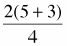2.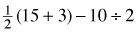3.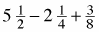4.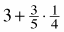5.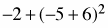6.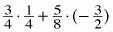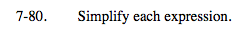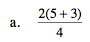Following the order of operations, first simplify the terms in parentheses, then the ones being multiplied or divided.
Lastly, combine any terms that are being added or subtracted.

4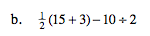See (a).

$3 \frac{5}{8}$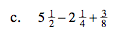You may find this expression easier to simplify by combining the whole numbers and the fractions separately.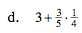See (a).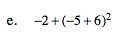First simplify the terms in parentheses, then the terms with exponents, and lastly combing any numbers that are added or subtracted.

−1

$- \frac{3}{4}$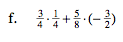See (a).